# Home Work Set # 3, Physics 217, Due: September 26, 2001

### Problem 1

a) Twelve equal charges, q, are situated at the corners of a regular 12-sided polygon (for instance, one on each numeral of a clock face). What is the net force on a test charge Q at the center?
b) Suppose one of the 12 q's is removed (the one at "6 o'clock"). What is the force on Q?
c) Now 13 equal charges, q, are placed at the corners of a regular 13-sided polygon. What is the force on a test charge Q at the center?
d) If one of the 13 q's is removed, what is the force on Q?

### Problem 2

Find the electric field a distance z above the center of a square loop (side s) carrying a uniform line charge λ (see Figure 1).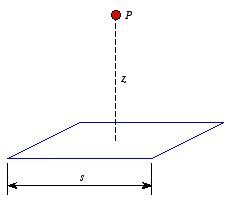Figure 1. Problem 2.

### Problem 3

Find the electric field a distance z above the center of a flat circular disc of radius R (see Figure 2), which carries a uniform surface charge σ. What does your formula give in the limit R → ∞. Also check the case z » R.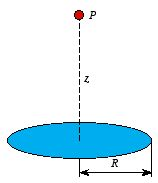Figure 2. Problem 3.

### Problem 4

Suppose the electric field in some region is found to be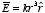.
a) Find the charge density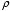.
b) Find the total charge contained in a sphere of radius R, centered at the origin. Do the calculation in two different ways.

### Problem 5

Use Gauss's law to find the electric field inside and outside a spherical shell of radius R, which carries a uniform surface charge density σ.

### Problem 6

A hollow spherical shell carries a charge density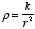in the region arb (see Figure 3).
a) Find the electric field in the region r < a.
b) Find the electric field in the region a < r < b.
c) Find the electric field in the region b < r.
d) Plot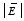as function of r.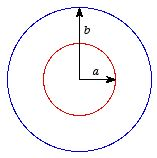Figure 3. Problem 6.

### Problem 7

An infinite plane slab, of thickness 2d, carries a uniform volume charge density(see Figure 4). Find the electric field, as a function of y, the distance from the center. Plot E versus y, calling E positive when it points in the +y direction and negative when it points in the -y direction.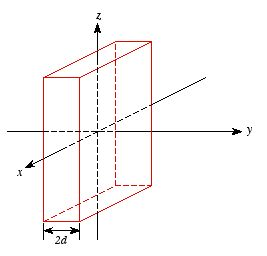Figure 4. Problem 7.